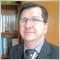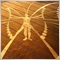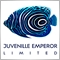# Discussion of article "The ZigZag Indicator: Fresh Approach and New Solutions" - page 2Moderator
2892

launic:

Formar o seu editor de ir verificar onde os indicadores são realmente armazenados

1. botão direito do mouse clique no arquivo de origem indicador

2. À esquerda, clique em Propriedades

3. Selecione a cópia valor Localização e colar na barra de endereços do Windows Explorer

Very Thanks Sir!

Now is working correct!51

Total newbie question but how can I use automate this EA to open positions based on the indicator???224

Line 74:   zzH[h]=(MqlZigZag)zz[i];  to     zzH[h].price=zz[i].value;

Line 80:   zzL[l]=(MqlZigZag)zz[i];    to    zzL[l].price=zz[i].value;

Also in the main program two_comment.mq5 the code block

//--- check for integrity of preliminary calculations - the CopyBuffer function results in an error thus err=-1 and it returns thus the rest of the program does not execute..

I just commented out the error checking or just set int err=1 so the program block does not run. and now the EA works for me.

//--- check for integrity of preliminary calculations

if(err<0)

{

//--- calculate the sums of deviations of the nodes from MA for ZigZag peaks

ArrayInitialize(sumHi,0.0);

for(int j=H-1; j>=0; j--)

{

for(int i=0; i<NUMBER_MA; i++)

{

err=CopyBuffer(handle_MA_H[i],0,Azz.zzH[j].t,1,MA);

if(err<0) return;

sumHi[i]+=Azz.zzH[j].price-MA;

//Print("No error! sumHi: ", sumHi[i]);

}

}

//--- calculate the sums of deviations of the nodes from MA for ZigZag troughs

Print("Error Number: ", err);

ArrayInitialize(sumLo,0.0);

for(int j=L-1; j>=0; j--)

{

for(int i=0; i<NUMBER_MA; i++)

{

err=CopyBuffer(handle_MA_L[i],0,Azz.zzL[j].t,1,MA);

if(err<0) return;

sumLo[i]+=MA-Azz.zzL[j].price;

}

}

}Moderator
17966